# Resources tagged with: Working systematically

Filter by: Content type:
Age range:
Challenge level:

### There are 342 results

Broad Topics > Thinking Mathematically > Working systematically### American Billions

##### Age 11 to 14 Challenge Level:

Play the divisibility game to create numbers in which the first two digits make a number divisible by 2, the first three digits make a number divisible by 3...### Product Sudoku

##### Age 11 to 16 Challenge Level:

The clues for this Sudoku are the product of the numbers in adjacent squares.### Multiples Sudoku

##### Age 11 to 14 Challenge Level:

Each clue in this Sudoku is the product of the two numbers in adjacent cells.### A First Product Sudoku

##### Age 11 to 14 Challenge Level:

Given the products of adjacent cells, can you complete this Sudoku?### A Mixed-up Clock

##### Age 7 to 11 Challenge Level:

There is a clock-face where the numbers have become all mixed up. Can you find out where all the numbers have got to from these ten statements?### The Moons of Vuvv

##### Age 7 to 11 Challenge Level:

The planet of Vuvv has seven moons. Can you work out how long it is between each super-eclipse?### Star Product Sudoku

##### Age 11 to 16 Challenge Level:

The puzzle can be solved by finding the values of the unknown digits (all indicated by asterisks) in the squares of the $9\times9$ grid.### How Old Are the Children?

##### Age 11 to 14 Challenge Level:

A student in a maths class was trying to get some information from her teacher. She was given some clues and then the teacher ended by saying, "Well, how old are they?"### Multiplication Squares

##### Age 7 to 11 Challenge Level:

Can you work out the arrangement of the digits in the square so that the given products are correct? The numbers 1 - 9 may be used once and once only.### Number Detective

##### Age 5 to 11 Challenge Level:

Follow the clues to find the mystery number.### Mystery Matrix

##### Age 7 to 11 Challenge Level:

Can you fill in this table square? The numbers 2 -12 were used to generate it with just one number used twice.### Curious Number

##### Age 7 to 11 Challenge Level:

Can you order the digits from 1-3 to make a number which is divisible by 3 so when the last digit is removed it becomes a 2-figure number divisible by 2, and so on?### Gabriel's Problem

##### Age 11 to 14 Challenge Level:

Gabriel multiplied together some numbers and then erased them. Can you figure out where each number was?### Ben's Game

##### Age 11 to 16 Challenge Level:

Ben, Jack and Emma passed counters to each other and ended with the same number of counters. How many did they start with?### Code Breaker

##### Age 7 to 11 Challenge Level:

This problem is based on a code using two different prime numbers less than 10. You'll need to multiply them together and shift the alphabet forwards by the result. Can you decipher the code?### Two Primes Make One Square

##### Age 7 to 11 Challenge Level:

Can you make square numbers by adding two prime numbers together?### Trebling

##### Age 7 to 11 Challenge Level:

Can you replace the letters with numbers? Is there only one solution in each case?### Number Daisy

##### Age 11 to 14 Challenge Level:

Can you find six numbers to go in the Daisy from which you can make all the numbers from 1 to a number bigger than 25?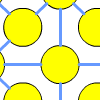### One to Fifteen

##### Age 7 to 11 Challenge Level:

Can you put the numbers from 1 to 15 on the circles so that no consecutive numbers lie anywhere along a continuous straight line?### Two and Two

##### Age 11 to 16 Challenge Level:

How many solutions can you find to this sum? Each of the different letters stands for a different number.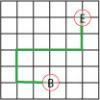### Journeys in Numberland

##### Age 7 to 11 Challenge Level:

Tom and Ben visited Numberland. Use the maps to work out the number of points each of their routes scores.### Cayley

##### Age 11 to 14 Challenge Level:

The letters in the following addition sum represent the digits 1 ... 9. If A=3 and D=2, what number is represented by "CAYLEY"?### ABC

##### Age 7 to 11 Challenge Level:

In the multiplication calculation, some of the digits have been replaced by letters and others by asterisks. Can you reconstruct the original multiplication?### Coded Hundred Square

##### Age 7 to 11 Challenge Level:

This 100 square jigsaw is written in code. It starts with 1 and ends with 100. Can you build it up?### Football Sum

##### Age 11 to 14 Challenge Level:

Find the values of the nine letters in the sum: FOOT + BALL = GAME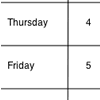### Today's Date - 01/06/2009

##### Age 5 to 11 Challenge Level:

What do you notice about the date 03.06.09? Or 08.01.09? This challenge invites you to investigate some interesting dates yourself.##### Age 11 to 14 Challenge Level:

If you take a three by three square on a 1-10 addition square and multiply the diagonally opposite numbers together, what is the difference between these products. Why?### Neighbours

##### Age 7 to 11 Challenge Level:

In a square in which the houses are evenly spaced, numbers 3 and 10 are opposite each other. What is the smallest and what is the largest possible number of houses in the square?### Factor Lines

##### Age 7 to 14 Challenge Level:

Arrange the four number cards on the grid, according to the rules, to make a diagonal, vertical or horizontal line.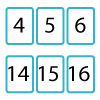### Twenty Divided Into Six

##### Age 7 to 11 Challenge Level:

Katie had a pack of 20 cards numbered from 1 to 20. She arranged the cards into 6 unequal piles where each pile added to the same total. What was the total and how could this be done?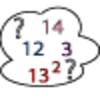### Seven Square Numbers

##### Age 7 to 11 Challenge Level:

Add the sum of the squares of four numbers between 10 and 20 to the sum of the squares of three numbers less than 6 to make the square of another, larger, number.### Making a Difference

##### Age 11 to 14 Challenge Level:

How many different differences can you make?### Spell by Numbers

##### Age 7 to 11 Challenge Level:

Can you substitute numbers for the letters in these sums?### Zargon Glasses

##### Age 7 to 11 Challenge Level:

Zumf makes spectacles for the residents of the planet Zargon, who have either 3 eyes or 4 eyes. How many lenses will Zumf need to make all the different orders for 9 families?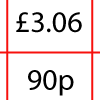### How Much Did it Cost?

##### Age 7 to 11 Challenge Level:

Use your logical-thinking skills to deduce how much Dan's crisps and ice-cream cost altogether.### Peaches Today, Peaches Tomorrow...

##### Age 11 to 14 Challenge Level:

A monkey with peaches, keeps a fraction of them each day, gives the rest away, and then eats one. How long can his peaches last?### An Introduction to Magic Squares

##### Age 7 to 16##### Age 11 to 14 Challenge Level:

Replace the letters with numbers to make the addition work out correctly. R E A D + T H I S = P A G E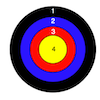### Dart Target

##### Age 7 to 11 Challenge Level:

This task, written for the National Young Mathematicians' Award 2016, invites you to explore the different combinations of scores that you might get on these dart boards.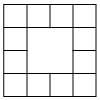### Prison Cells

##### Age 7 to 11 Challenge Level:

There are 78 prisoners in a square cell block of twelve cells. The clever prison warder arranged them so there were 25 along each wall of the prison block. How did he do it?### Being Resourceful - Primary Number

##### Age 5 to 11 Challenge Level:

Number problems at primary level that require careful consideration.##### Age 11 to 16 Challenge Level:

The items in the shopping basket add and multiply to give the same amount. What could their prices be?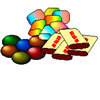### The Puzzling Sweet Shop

##### Age 5 to 11 Challenge Level:

There were chews for 2p, mini eggs for 3p, Chocko bars for 5p and lollypops for 7p in the sweet shop. What could each of the children buy with their money?##### Age 7 to 11 Challenge Level:

Can you put plus signs in so this is true? 1 2 3 4 5 6 7 8 9 = 99 How many ways can you do it?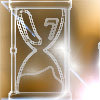### Two Egg Timers

##### Age 7 to 11 Challenge Level:

You have two egg timers. One takes 4 minutes exactly to empty and the other takes 7 minutes. What times in whole minutes can you measure and how?### Magic Vs

##### Age 7 to 11 Challenge Level:

Can you put the numbers 1-5 in the V shape so that both 'arms' have the same total?### A-magical Number Maze

##### Age 7 to 11 Challenge Level:

This magic square has operations written in it, to make it into a maze. Start wherever you like, go through every cell and go out a total of 15!### All the Digits

##### Age 7 to 11 Challenge Level:

This multiplication uses each of the digits 0 - 9 once and once only. Using the information given, can you replace the stars in the calculation with figures?### Making Maths: Double-sided Magic Square

##### Age 7 to 14 Challenge Level:

Make your own double-sided magic square. But can you complete both sides once you've made the pieces?### Cinema Problem

##### Age 11 to 14 Challenge Level:

A cinema has 100 seats. Show how it is possible to sell exactly 100 tickets and take exactly £100 if the prices are £10 for adults, 50p for pensioners and 10p for children.